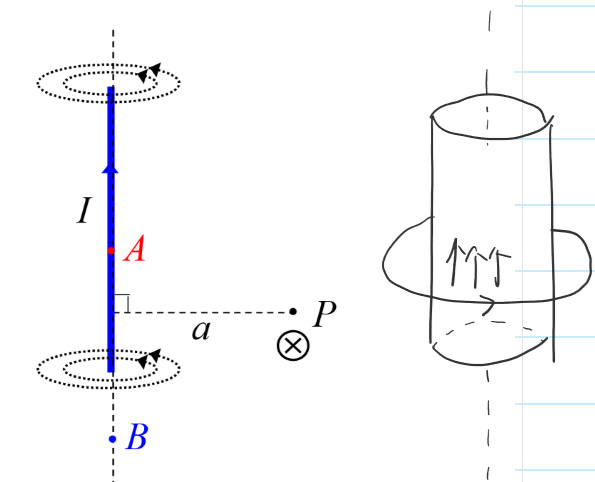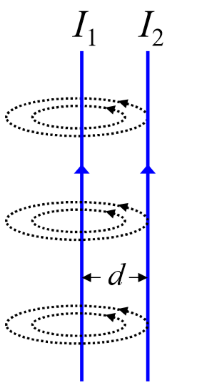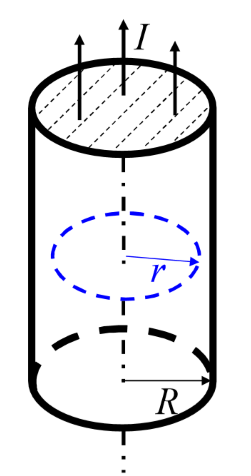# Physics Speedrun - Magnetic Field

Magnetism is the interaction of clectric currents or moving charges. Magnetic field is

1. Created by / interacts on currents or moving charges
2. Closed field lines without beginning or end

## Ampere Force

Magnetic field exerts a force on a current, called Ampere forece. In uniform field:

The magnetic field is defind by the formula . SI Unit for : Tesla

General case: nonuniform , curved wire:

In uniform field, it is equivalant to a stright wire from A to B.

Magnetic field freated by current

### Magnetic Dipole

Total force is zero, but torque on the loop:

Magnetic dipole moment:

Direction of is defined by the right-hand rule.

Torque:

Valid for any plane current loop. A small circular current is called a magnetic dipole.

For a loops coil / solenoid:

Orbital angular momentum:

### Lorentz Force

Magnetic field exerts a force on a moving charge:

Lorentz force does not do work on the charge.

When , , free motion.

When , the point charge do a uniform circular motion.

The charge moves in a helix.

Lorentz equation: Total force on a charge in electromagnet field

## Source of Magnetic Field

### Biot-Savart Law

Magnetic equivalant to Coulumb's law. Magnetic field due to an infinitesimal current:

Permeability of free space:

Direction: right-hand rule.

Total magnetic field due to a full current:

Infinite straight current:No magnetic field at A or B. Also no field inside the cylinder when all the current is on the surface.Force between parallel wires

per unit length:

Magnetic dipole moment:

### Gauss's Law for Magnetic Field

Magnetic flux:

Gauss's law for magnetic field:

Magnetic field lines are closed lines without beginning or end.

### Ampere's Law

Magnetic field is produced by all currents:

Magnetic field is not a conservative field:

Maxwell equations for steady magnetic field and electrostatic field:Proper integral path for calculating magnetic field of a cylinderical current